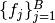# Sampler¶

Each of thesampling functions of the Mamba Gibbs sampling scheme is implemented as a `Sampler` type object, whose fields are summarized herein. The `eval` field is an anonymous function defined as

```function(model::Mamba.Model, block::Integer)
```

where `model` contains all model nodes, and `block` is an index identifying the corresponding sampling function in a vector of all samplers for the associated model. Through the arguments, all model nodes and fields can be accessed in the body of the function. The function may return an updated sample for the nodes identified in its `params` field. Such a return value can be a structure of the same type as the node if the block consists of only one node, or a dictionary of node structures with keys equal to the block node symbols if one or more. Alternatively, a value of `nothing` may be returned. Return values that are not `nothing` will be used to automatically update the node values and propagate them to dependent nodes. No automatic updating will be done if `nothing` is returned.

## Declaration¶

`type Sampler`

## Fields¶

• `params::Vector{Symbol}` : symbols of stochastic nodes in the block being updated by the sampler.
• `eval::Function` : a sampling function that updates values of the `params` nodes.
• `tune::Dict{String,Any}` : any tuning parameters needed by the sampling function.
• `targets::Vector{Symbol}` : symbols of `Dependent` nodes that depend on and whose states must be updated after `params`. Elements of `targets` are topologically sorted so that a given node in the vector is conditionally independent of subsequent nodes, given the previous ones.

## Constructor¶

`Sampler`(params::Vector{Symbol}, expr::Expr, tune::Dict=Dict())

Construct a `Sampler` object that defines a sampling function for a block of stochastic nodes.

Arguments

• `params` : symbols of nodes that are being block-updated by the sampler.
• `expr` : a quoted expression that makes up the body of the sampling function whose definition is described above.
• `tune` : tuning parameters needed by the sampling function.

Value

Returns a `Sampler` type object.

Example

See the Model Specification section of the tutorial.

## Methods¶

`show`(s::Sampler)

Write a text representation of the defined sampling function to the current output stream.

`showall`(s::Sampler)

Write a verbose text representation of the defined sampling function to the current output stream.